• 有源低通滤波器设计
千次阅读
2022-03-10 09:33:20

# 【 1. 一阶 】

• 积分运算电路具有低通特性，但是当频率趋于零时电压放大倍数的数值趋于无穷大，其幅频特性如下图b中虚线所示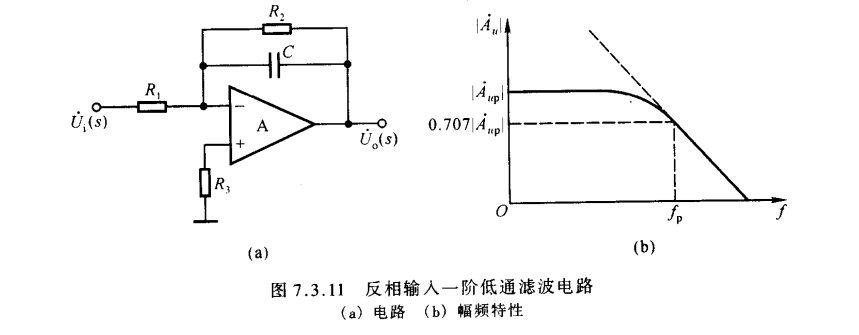• 由前面的分析可知，通带放大倍数决定于由电阻组成的负反馈网络，故在积分运算电路中电容上并联一个电阻，可得到上图a所示的反相输入一阶低通滤波电路.

• 电路的 传递函数
A u ( s ) = − R 2 / / 1 s C R 1 = − R 2 R 1 ⋅ 1 1 + s R 2 C A_u(s)=-\frac{R_2//\frac{1}{sC}}{R_1}=-\frac{R_2}{R_1}·\frac{1}{1+sR_2C}

• 令输入信号频率等于零（ s = j w = 2 π f = 0 s=jw=2\pi f=0 ），可得 通带放大倍数
A u p = − R 2 R 1 A_{up}=-\frac{R_2}{R_1}

• 用 jw 代替 s，令 f 0 = 1 2 π R 2 C f_0=\frac{1}{2\pi R_2C} ，得出 电压放大倍数
A u = A u p 1 + j f f 0 A_u=\frac{A_{up} }{1+j\frac{f}{f_0} }

• 通带截止频率
f = f 0 f=f_0
幅频特性曲线如上图b中实线所示

# 【 2. 二阶 】

• 与同相输人电路类似，增加RC环节，可以使滤波器的过渡带变窄， 衰减斜率的值加大，电路如下图所示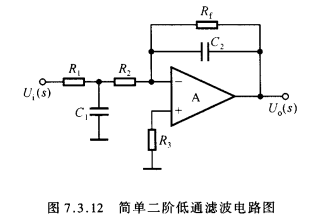• 为了改善 f 0 f_0 附近的频率特性，也可采用压控电压源二阶滤波器相似的方法，即多路反馈的方法，电路如下图所示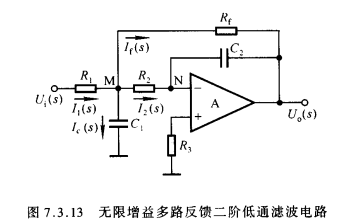• 在上图所示电路中，当 f = 0 f=0 时， C 1 C_1 C 2 C_2 均开路，故 通带放大倍数
A u p = − R f R 1 A_{up}=-\frac{R_f}{R_1}
• M 点的电流方程
I 1 ( s ) = I f ( s ) + I 2 ( s ) + I C ( s ) 故   U i ( s ) − U M ( s ) R 1 = U M ( s ) − U o ( s ) R f + U M ( s ) R 2 + U M ( s ) s C 1 I_1(s)=I_f(s)+I_2(s)+I_C(s)\\ 故\ \frac{U_i(s)-U_M(s)}{R_1}=\frac{U_M(s)-U_o(s)}{R_f}+\frac{U_M(s)}{R_2}+U_M(s)sC_1
• 其中
U M ( s ) R 2 = − U o ( s ) 1 s C 2 故   U o ( s ) = − 1 s R 2 C 2 ⋅ U M ( s ) \frac{U_M(s)}{R_2}=-\frac{U_o(s)}{\frac{1}{sC_2}}\\ 故 \ U_o(s)=-\frac{1}{sR_2C_2}·U_M(s)
• 联立上三式，得到 传递函数
A u ( s ) = A u p ( s ) 1 + s C 2 R 2 R f ( 1 R 1 + 1 R 2 + 1 R f ) + s 2 C 1 C 2 R 2 R f A_u(s)=\frac{A_{up}(s)}{1+sC_2R_2R_f(\frac{1}{R_1}+\frac{1}{R_2}+\frac{1}{R_f})+s^2C_1C_2R_2R_f}
• 与压控电源二阶同相低通滤波电路传递函数对比，可得
f 0 = 1 2 π C 1 C 2 R 2 R f Q = ( R 1 / / R 2 / / R f ) C 1 R 2 R f C 2 f_0=\frac{1}{2\pi \sqrt{C_1C_2R_2R_f}}\\ Q=(R_1//R_2//R_f)\sqrt{\frac{C_1}{R_2R_fC_2}}
• 从传递函数式子的分母可以看出，滤波器不会因通带放大倍数数值过大而产生自激振荡。因为上图所示电路中的运放可看成理想运放，即可认为其增益无穷大，故称该电路为 无限增益多路反馈滤波电路
• 当多个低通滤波器串联起来时，就可得到高阶低通滤波器，下图所示为四阶低通滤波器的方框图。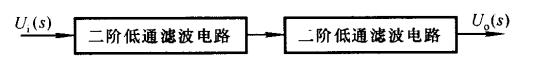滤波器
更多相关内容
• 自己的电压跟随低通滤波器和无限增益低通滤波器的计算器，能计算的截止频率和品质因数，电路一目了然
• 给出了巴特沃兹有源低通滤波器设计方法和设计实例
• 基于MATLAB的有源低通滤波器设计与实现.pdf
• 数字信号处理课程设计代码，大报告详见https://blog.csdn.net/weixin_42845306/article/details/117033419 上传之后才发现缺失一部分代码，不好意思，这就补上 function [Bz,Az]=lvboqi() fs=100;%采样频率 T=1/fs;...
• 其中，有源低通滤波电路由集成运放和无源元件电阻和电容构成。它的功能是允许从零到某个截止频率的信号无衰减地通过，而对其他频率的信号有抑制作用，有源低通滤波电路可以用来滤除高频干扰信号。
• ## 二阶有源低通滤波器设计

万次阅读 多人点赞 2016-04-13 18:58:45
滤波一般可分为有源滤波和无源滤波, 有源滤波可以使幅频特性比较陡峭, 而无源滤波设计简单易行, 但幅频特性不如滤波器, 而且体积较大。从滤波器阶数可分为一阶和高阶, 阶数越高, 幅频特性越陡峭。高阶滤波器通常可由...

滤波器是一种使用信号通过而同时抑制无用频率信号的电子装置, 在信息处理、数据传送和抑制干扰等自动控制、通信及其它电子系统中应用广泛。滤波一般可分为有源滤波和无源滤波, 有源滤波可以使幅频特性比较陡峭, 而无源滤波设计简单易行, 但幅频特性不如滤波器, 而且体积较大。从滤波器阶数可分为一阶和高阶, 阶数越高, 幅频特性越陡峭。高阶滤波器通常可由一阶和二阶滤波器级联而成。采用集成运放构成的RC 有源滤波器具有输入阻抗高,输出阻抗低, 可提供一定增益, 截止频率可调等特点 。压控电压源型二阶低通滤波电路是有源滤波电路的重要一种, 适合作为多级放大器的级联。本文根据实际要求设计一种压控电压源型二阶有源低通滤波电路, 采用E仿真软件Mu ltisim10对压控电压源型二阶有源低通滤波电路进行仿真分析、调试, 从而实现电路的优化设计。

1.设计分析

二阶有源低通滤波器典型结构如下图所示：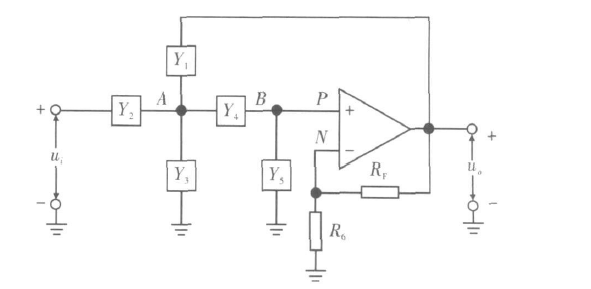其中, Y1 ~ Y5 为导纳, 考虑到Up = UN , 根据KCL 可得到如下：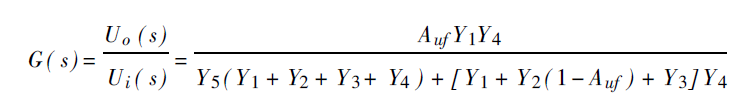Auf = 1+ RF /R 6。只要适当选择Yi, 就可以构成低通、高通、带通等有源滤波器。设Y1 = 1 /R 1, Y2 = sC1, Y3 = 0, Y4 = 1 /R 2, Y5 =
sC2, 将其代入式( 1)中, 得到压控电压源型二阶有源低通滤波器的传递函数为：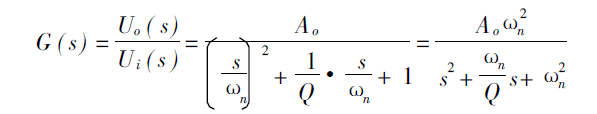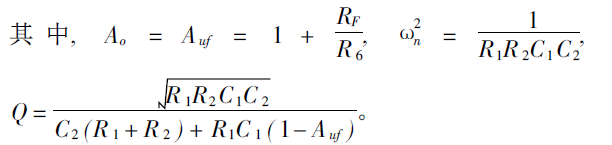这是二阶低通滤波器传递函数的典型表达式。其中, Xn 为特征角频率, Q 称为等效品质因数。

2.动手设计一款带宽在340HZ的有源滤波器，电容10nf，放大倍数2.

为设计方便选取R1 = R2 = R, C1 = C2 = C, 则通带截止频率为f 0 = fn =1/2πrc,c=10nf.推出R=47Kohm

电路如下所示：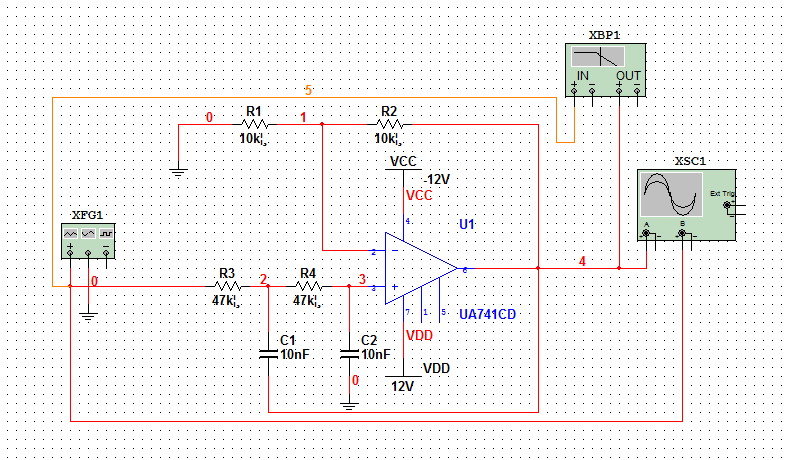波形如下所示，100hz正弦波：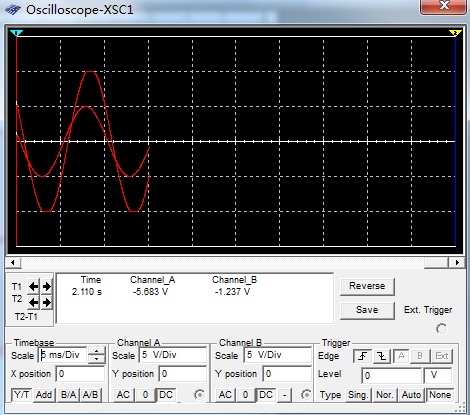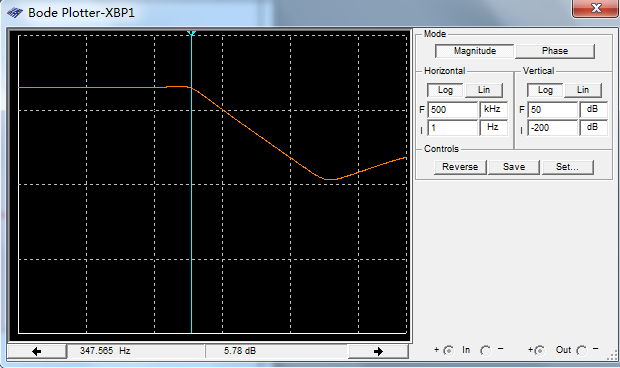展开全文• 2．四种滤波器的传递函数和实验模拟电路如图4-2 所示：(a)无源低通滤波器 (b)有源低通滤波器(c) 无源高通滤波器 (d)有源高通滤波器(e)无源带通滤波器 (f)有源带通滤波器(g)无源带阻滤波器 (h)有源带阻滤波器 ...
• 设计二阶低通滤波器，电源电压± 5V，要求完成下面的技术指标： a. 滤波器的截止频率fH ≤100HZ； b. 通带电压增益Au ≥ 1　；
• 设计有源低通二阶滤波器(又称Sallen-Key 低通滤波器)使用公式法计算得到各参数,本设计从运算放大器的输出到同相端输入间引入一个负反馈构建而成。本文详细叙述了如何用计算法设计有源低通二阶滤波器的方法，并用...
• 有源带通滤波器有源低通滤波器有源高通滤波器有源陷波器有源谐振滤波器5个Multisim源码文件
• 该电路不含任何电阻，仅使用1个新的多输出电流差分级联跨导放大器和若干接地电容，能实现任意阶低通滤波器功能。使用CADENCE进行设计和调试，电路芯片面积仅0.04 mm2，仿真功耗仅4.18 mW，最大3 dB截止频率500 MHz。...
• 模拟电子电路中的众多仿真电路资源，MULTISM10.0版本的，都是运行过得电路非常好用。二阶有源低通滤波器的仿真分析。
• 有源低通滤波器 基于proteus的 模拟电子技术 课程设计 1000hz q=0.707proteus
• sC2, 将其代入式( 1)中, 得到压控电压源型二阶有源低通滤波器的传递函数为： 这是二阶低通滤波器传递函数的典型表达式。其中, Xn 为特征角频率, Q 称为等效品质因数。 2.动手设计一款带宽在340HZ的有源...
                                        <link rel="stylesheet" href="https://csdnimg.cn/release/phoenix/template/css/ck_htmledit_views-e2445db1a8.css">
<div class="htmledit_views">


滤波器是一种使用信号通过而同时抑制无用频率信号的电子装置, 在信息处理、数据传送和抑制干扰等自动控制、通信及其它电子系统中应用广泛。滤波一般可分为有源滤波和无源滤波, 有源滤波可以使幅频特性比较陡峭, 而无源滤波设计简单易行, 但幅频特性不如滤波器, 而且体积较大。从滤波器阶数可分为一阶和高阶, 阶数越高, 幅频特性越陡峭。高阶滤波器通常可由一阶和二阶滤波器级联而成。采用集成运放构成的RC 有源滤波器具有输入阻抗高,输出阻抗低, 可提供一定增益, 截止频率可调等特点 。压控电压源型二阶低通滤波电路是有源滤波电路的重要一种, 适合作为多级放大器的级联。本文根据实际要求设计一种压控电压源型二阶有源低通滤波电路, 采用E仿真软件Mu ltisim10对压控电压源型二阶有源低通滤波电路进行仿真分析、调试, 从而实现电路的优化设计。

1.设计分析

二阶有源低通滤波器典型结构如下图所示：其中, Y1 ~ Y5 为导纳, 考虑到Up = UN , 根据KCL 可得到如下：Auf = 1+ RF /R 6。只要适当选择Yi, 就可以构成低通、高通、带通等有源滤波器。设Y1 = 1 /R 1, Y2 = sC1, Y3 = 0, Y4 = 1 /R 2, Y5 =
sC2, 将其代入式( 1)中, 得到压控电压源型二阶有源低通滤波器的传递函数为：这是二阶低通滤波器传递函数的典型表达式。其中, Xn 为特征角频率, Q 称为等效品质因数。

2.动手设计一款带宽在340HZ的有源滤波器，电容10nf，放大倍数2.

为设计方便选取R1 = R2 = R, C1 = C2 = C, 则通带截止频率为f 0 = fn =1/2πrc,c=10nf.推出R=47Kohm

电路如下所示：波形如下所示，100hz正弦波：展开全文滤波器
• 有源低通滤波器实验电路multisim源文件，multisim10及以上版本可以正常打开仿真，是教材上的电路，可以直接仿真，方便大家学习。multisim
• 基于Multisim 8的有源低通滤波器仿真设计研究，林文剑，王涛，本文简要介绍了Multisim 8仿真软件的特点，给出了有源低通滤波器仿真设计的一般方法，分析了一阶和二阶有源低通滤波电路的性能。在��
• 　一、无差拍 SVPWM 的有源滤波器设计　有源电力滤波器（AcTIve Power Filter，APF）作为一种用于动态抑制谐波的电力电子装置，其能够同时补偿多次谐波电流，能实时控制、自动跟踪非线性电流并加以控制，有较快的...
• 可得实际增益为增益值是频率的函数，在低频区ω极小， RωC << 1，AV(ω) = 1讯号可通；在高频区ω极大， RωC >> 1，AV(ω) = 0信号不通。 RωC = 1时是通与不通的临界点，...低通有源滤波器在低频区的...可得实际增益为增益值是频率的函数，在低频区ω极小， RωC << 1，AV(ω) = 1讯号可通；在高频区ω极大， RωC >> 1，AV(ω) = 0信号不通。 RωC = 1时是通与不通的临界点，此时的频率定义为截止频率：

。图4所示RC低通滤波电路的增益随频率的变化是缓慢的，故其不是一个好的滤波电

路。图5所示是低通有源滤波器，它的增益显示在图6。低通有源滤波器在低频区的增益为：

VO/VI=(R1+R2)/R2

其推导如下：在低频区RC串联之电位降都在电容，故Vin = VC = Vp。见图5，因负回馈，电路在线性工作区，于是我们有关系式：

阻R2之电位降相同，又流过R1与R2之电流相同均为I，故得到，可知电容C之电位降与电

在高频区RC串联之电位降都在电阻，故VC = Vp = 0。因负回馈，电路在线性工作区，于是有关系式：，得到R2之电位降为0，I = 0，V0 = 0。图3 RC低通无源滤波电路

展开全文• 本文给大家分享了有源低通滤波器(LM102)电路图。
• 详细的讲述了CMOS有源二阶低通滤波器设计方法和流程，并且解决了低通滤波器不稳定的问题。滤波器
• 有源一阶低通滤波电路实验电路multisim源文件，multisim10及以上版本可以正常打开仿真，是教材上的电路，可以直接仿真，方便大家学习。multisim
• 贝塞尔有源低通滤波器元件值如下表所示： 　来源:ks99
• 常见的多级低通有源滤波器的增益排序方法是把大部分乃至全部增益放在第一级。如果只考虑要降低低频的输入参考噪声，这是正确的设计方法。然而，其它的几种考虑因素可能会使您改变这种增益排序，以实现更为出色的实施...信号调理
• 设计一种压控电压源型二阶有源低通滤波电路，并利用Multisim10仿真软件对电路的频率特性、特征参量等进...信号调理...